# Existence of solutions to strongly damped plate or beam equations

## Abstract

In this paper, we study a strongly damped plate or beam equation. By using spatial sequence techniques and energy estimate methods, we obtain an existence theorem of the solution to abstract strongly damped plate or beam equation and to a nonlinear plate or beam equation.

MSC:35L05, 35L20, 35D30, 35D35.

## 1 Introduction

We consider the following nonlinear strongly damped plate or beam equation:

$\left\{\begin{array}{c}\frac{{\partial }^{2}u}{\partial {t}^{2}}-k\frac{\partial \mathrm{△}u}{\partial t}=f\left(x,{\mathrm{△}}^{2}u\right)+g\left(x,u,Du,{D}^{2}u,{D}^{3}u\right),\phantom{\rule{1em}{0ex}}k>0,\hfill \\ u{|}_{\partial \mathrm{\Omega }}=\mathrm{△}u{|}_{\partial \mathrm{\Omega }}=0,\hfill \\ u\left(x,0\right)=\phi ,\phantom{\rule{2em}{0ex}}{u}_{t}\left(x,0\right)=\psi ,\hfill \end{array}$
(1.1)

where Δ is the Laplacian operator, Ω denotes an open bounded set of ${R}^{N}$ ($N=1,2$) with a smooth boundary Ω and u denotes a vertical displacement at $\left(x,t\right)$.

It is well known that flexible structures like suspension bridges or overhead power transmission lines can be subjected to oscillations due to various causes. Simple models for such oscillations are described with second- and fourth-order partial differential equations as can be seen for example in . The problem (1.1) can be applied in the mechanics of elastic constructions for the study of equilibrium forms of the plate and beam, which has a long history. The abstract theory of Eq. (1.1) was investigated by several authors .

The main objective of this article is to find proper conditions on f and g to ensure the existence of solutions of Eq. (1.1). This article uses the spatial sequence techniques, each side of the equation to be treated in different spaces, which is an important way to get more extensive and wonderful results.

The outline of the paper is as follows. In Section 2 we provide an essential definition and lemma of solutions to abstract equations from . In Section 3, we give an existence theorem of solutions to abstract strongly damped plate or beam equations. In Section 4.10, we present the main result and its proof.

## 2 Preliminaries

We introduce two spatial sequences:

$\left\{\begin{array}{c}X\subset {H}_{3}\subset {X}_{2}\subset {X}_{1}\subset H,\hfill \\ {X}_{2}\subset {H}_{2}\subset {H}_{1}\subset H,\hfill \end{array}$
(2.1)

where H, ${H}_{1}$, ${H}_{2}$, ${H}_{3}$ are Hilbert spaces, X is a linear space, and ${X}_{1}$, ${X}_{2}$ are Banach spaces. All embeddings of (2.1) are dense. Let

$\left\{\begin{array}{c}L:X\to {X}_{1}\phantom{\rule{1em}{0ex}}\text{be one-one dense linear operator},\hfill \\ {〈Lu,v〉}_{H}={〈u,v〉}_{{H}_{1}},\phantom{\rule{1em}{0ex}}\mathrm{\forall }u,v\in X.\hfill \end{array}$
(2.2)

Furthermore, L has eigenvectors $\left\{{e}_{k}\right\}$ satisfying

$L{e}_{k}={\lambda }_{k}{e}_{k}\phantom{\rule{1em}{0ex}}\left(k=1,2,\dots \right),$
(2.3)

and $\left\{{e}_{k}\right\}$ constitutes a common orthogonal basis of H and ${H}_{3}$.

We consider the following abstract equation:

$\left\{\begin{array}{c}\frac{{d}^{2}u}{d{t}^{2}}+k\frac{d}{dt}\mathcal{L}u=G\left(u\right),\phantom{\rule{1em}{0ex}}k>0,\hfill \\ u\left(0\right)=\phi ,\phantom{\rule{2em}{0ex}}{u}_{t}\left(0\right)=\psi ,\hfill \end{array}$
(2.4)

where $G:{X}_{2}×{R}^{+}\to {X}_{1}^{\ast }$ is a mapping, ${R}^{+}=\left[0,\mathrm{\infty }\right)$ and $\mathcal{L}:{X}_{2}\to {X}_{1}$ is a bounded linear operator satisfying

${〈\mathcal{L}u,Lv〉}_{H}={〈u,v〉}_{{H}_{2}},\phantom{\rule{1em}{0ex}}\mathrm{\forall }u,v\in {X}_{2}.$
(2.5)

Definition 2.1

We say $u\in {W}_{\mathrm{loc}}^{1,\mathrm{\infty }}\left(\left(0,\mathrm{\infty }\right),{H}_{1}\right)\cap {L}_{\mathrm{loc}}^{\mathrm{\infty }}\left(\left(0,\mathrm{\infty }\right),{X}_{2}\right)$ is a global weak solution of Eq. (2.4) provided that $\left(\phi ,\psi \right)\in {X}_{2}×{H}_{1}$

${〈{u}_{t},v〉}_{H}+k{〈\mathcal{L}u,v〉}_{H}={\int }_{0}^{t}〈G\left(u\right),v〉\phantom{\rule{0.2em}{0ex}}dt+{〈\psi ,v〉}_{H}+k{〈\mathcal{L}\phi ,v〉}_{H},$
(2.6)

for all $v\in {X}_{1}$ and $0\le t<\mathrm{\infty }$.

Lemma 2.2

Let$u\in {L}_{\mathrm{loc}}^{p}\left(\left(-\mathrm{\infty },\mathrm{\infty }\right),X\right)$, X be a Banach space. If${u}_{h}=\frac{1}{h}{\int }_{h}^{t+h}u\left(s\right)\phantom{\rule{0.2em}{0ex}}ds$ ($0<|h|<1$), then$\left\{{u}_{h}\right\}\in {L}_{\mathrm{loc}}^{p}\left(\left(-\mathrm{\infty },\mathrm{\infty }\right),X\right)$, satisfying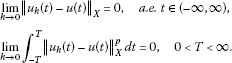## 3 Existence theorem of abstract equation

Let $G=A+B:{X}_{2}×{R}^{+}\to {X}_{1}^{\ast }$. Assume:

(A1) There is a ${C}^{1}$ functional $F:{X}_{2}\to {R}^{1}$ such that

$〈Au,Lv〉=〈-DF\left(u\right),v〉,\phantom{\rule{1em}{0ex}}\mathrm{\forall }u,v\in X.$
(3.1)

(A2) Functional $F:{X}_{2}\to {R}^{1}$ is coercive, i.e.,

$F\left(u\right)\to \mathrm{\infty },\phantom{\rule{1em}{0ex}}⇔\phantom{\rule{1em}{0ex}}{\parallel u\parallel }_{{X}_{2}}\to \mathrm{\infty }.$
(3.2)

(A3) B satisfies

$|〈Bu,Lv〉|\le CF\left(u\right)+\frac{k}{2}{\parallel v\parallel }_{{H}_{2}}^{2}+g\left(t\right),\phantom{\rule{1em}{0ex}}\mathrm{\forall }u,v\in X,$
(3.3)

for $g\in {L}_{\mathrm{loc}}^{1}\left(0,\mathrm{\infty }\right)$.

Theorem 3.1 If$G:{X}_{2}×{R}^{+}\to {X}_{1}^{\ast }$is bounded and continuous, and DF is monotone, i.e.,

$〈DF\left({u}_{1}\right)-DF\left({u}_{2}\right),{u}_{1}-{u}_{2}〉\ge 0,\phantom{\rule{1em}{0ex}}\mathrm{\forall }{u}_{1},{u}_{2}\in {X}_{2},$
(3.4)

then, for all$\left(\phi ,\psi \right)\in {X}_{2}×{H}_{1}$, the following assertions hold.

1. (1)

If $G=A$ satisfies (A 1) and (A 2), then Eq. (2.4) has a global weak solution

$u\in {W}^{1,\mathrm{\infty }}\left(\left(0,\mathrm{\infty }\right),{H}_{1}\right)\cap {W}^{1,2}\left(\left(0,\mathrm{\infty }\right),{H}_{2}\right)\cap {L}^{\mathrm{\infty }}\left(\left(0,\mathrm{\infty }\right),{X}_{2}\right).$
(3.5)
2. (2)

If $G=A+B$ satisfies (A 1)-(A 3), and ${u}_{n}{⇀}^{\ast }{u}_{0}$ in ${L}^{\mathrm{\infty }}\left(\left(0,T\right),{X}_{2}\right)$ such that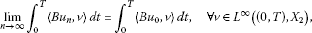(3.6)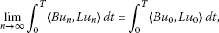(3.7)

then Eq. (2.4) has a global weak solution

$u\in {W}_{\mathrm{loc}}^{1,\mathrm{\infty }}\left(\left(0,\mathrm{\infty }\right),{H}_{1}\right)\cap {W}_{\mathrm{loc}}^{1,2}\left(\left(0,\mathrm{\infty }\right),{H}_{2}\right)\cap {L}_{\mathrm{loc}}^{\mathrm{\infty }}\left(\left(0,\mathrm{\infty }\right),{X}_{2}\right).$
(3.8)
1. (3)

Furthermore, if $G=A+B$ satisfies

$|〈Gu,v〉|\le CF\left(u\right)+\frac{1}{2}{\parallel v\parallel }_{H}^{2}+g\left(t\right),$
(3.9)

for$g\in {L}^{1}\left(0,T\right)$, then$u\in {W}_{\mathrm{loc}}^{2,2}\left(\left(0,\mathrm{\infty }\right);H\right)$.

Proof Let $\left\{{e}_{k}\right\}\subset X$ be a common orthogonal basis of H and ${H}_{3}$, satisfying (2.3). Set

$\left\{\begin{array}{c}{X}_{n}=\left\{\sum _{i=1}^{n}{\alpha }_{i}{e}_{i}|{\alpha }_{i}\in {R}^{1}\right\},\hfill \\ {\stackrel{˜}{X}}_{n}=\left\{\sum _{j=1}^{n}{\beta }_{j}\left(t\right){e}_{j}|{\beta }_{j}\in {C}^{2}\left[0,\mathrm{\infty }\right)\right\}.\hfill \end{array}$
(3.10)

Clearly, $L{X}_{n}={X}_{n}$, $L{\stackrel{˜}{X}}_{n}={\stackrel{˜}{X}}_{n}$.

By using Galerkin method, there exists ${u}_{n}\in {C}^{2}\left(\left[0,\mathrm{\infty }\right),{X}_{n}\right)$ satisfying

$\left\{\begin{array}{c}{〈\frac{d{u}_{n}}{dt},v〉}_{H}+k{〈\mathcal{L}{u}_{n},v〉}_{H}={\int }_{0}^{t}〈G\left({u}_{n}\right),v〉\phantom{\rule{0.2em}{0ex}}dt+{〈{\psi }_{n},v〉}_{H}+k{〈\mathcal{L}{\phi }_{n},v〉}_{H},\hfill \\ {u}_{n}\left(0\right)={\phi }_{n},\phantom{\rule{2em}{0ex}}{u}_{n}^{\prime }\left(0\right)={\psi }_{n},\hfill \end{array}$
(3.11)

for $\mathrm{\forall }v\in {X}_{n}$, and

${\int }_{0}^{t}\left[{〈\frac{{d}^{2}{u}_{n}}{{d}^{2}t},v〉}_{H}+k{〈\mathcal{L}\frac{d{u}_{n}}{dt},v〉}_{H}\right]\phantom{\rule{0.2em}{0ex}}dt={\int }_{0}^{t}〈G{u}_{n},v〉\phantom{\rule{0.2em}{0ex}}dt$
(3.12)

for $\mathrm{\forall }v\in {\stackrel{˜}{X}}_{n}$.

Firstly, we consider $G=A$. Let $v=\frac{d}{dt}L{u}_{n}$ in (3.12). Taking into account (2.2)and (3.1), it follows that

$\begin{array}{rcl}0& =& {\int }_{0}^{t}\left[\frac{1}{2}\frac{d}{dt}{〈\frac{d{u}_{n}}{dt},\frac{d{u}_{n}}{dt}〉}_{{H}_{1}}+k{〈\frac{d{u}_{n}}{dt},\frac{d{u}_{n}}{dt}〉}_{{H}_{2}}+〈DF\left({u}_{n}\right),\frac{d{u}_{n}}{dt}〉\right]\phantom{\rule{0.2em}{0ex}}dt\\ =& \frac{1}{2}{\parallel \frac{d{u}_{n}}{dt}\parallel }_{{H}_{1}}^{2}-\frac{1}{2}{\parallel {\psi }_{n}\parallel }_{{H}_{1}}^{2}+k{\int }_{0}^{t}{\parallel \frac{d{u}_{n}}{dt}\parallel }_{{H}_{2}}^{2}\phantom{\rule{0.2em}{0ex}}dt+F\left({u}_{n}\right)-F\left({\phi }_{n}\right).\end{array}$

We get

$\frac{1}{2}{\parallel \frac{d{u}_{n}}{dt}\parallel }_{{H}_{1}}^{2}+k{\int }_{0}^{t}{\parallel \frac{d{u}_{n}}{dt}\parallel }_{{H}_{2}}^{2}dt+F\left({u}_{n}\right)=F\left({\phi }_{n}\right)+\frac{1}{2}{\parallel {\psi }_{n}\parallel }_{{H}_{1}}^{2}.$
(3.13)

Let $\phi \in {H}_{3}$. From (2.1) and (2.2), it is known that $\left\{{e}_{n}\right\}$ is an orthogonal basis of ${H}_{1}$. We find that ${\phi }_{n}\to \phi$ in ${H}_{3}$, and ${\psi }_{n}\to \psi$ in ${H}_{1}$. From that ${H}_{3}\subset {X}_{2}$ is an imbedding, it follows that

(3.14)

From (3.2), (3.13) and (3.14), we obtain

Let

(3.15)

which implies that ${u}_{n}\to {u}_{0}$ in ${W}^{1,2}\left(\left(0,\mathrm{\infty }\right),H\right)$ is uniformly weakly convergent from that ${H}_{2}\subset H$ is a compact imbedding.

According to (2.2), (2.4), (2.5) and (3.4), we obtain that

$\begin{array}{rcl}0& \ge & {\int }_{0}^{t}〈DF\left(v\right)-DF\left({u}_{n}\right),{u}_{n}-v〉\phantom{\rule{0.2em}{0ex}}d\tau \\ =& {\int }_{0}^{t}〈Av,Lv-L{u}_{n}〉\phantom{\rule{0.2em}{0ex}}d\tau +{\int }_{0}^{t}〈A{u}_{n},L{u}_{n}-Lv〉\phantom{\rule{0.2em}{0ex}}d\tau \\ =& {\int }_{0}^{t}〈Av,Lv-L{u}_{n}〉\phantom{\rule{0.2em}{0ex}}d\tau +{\int }_{0}^{t}{〈\frac{{d}^{2}{u}_{n}}{d{t}^{2}}+k\frac{d}{dt}\mathcal{L}{u}_{n},L{u}_{n}-Lv〉}_{H}\phantom{\rule{0.2em}{0ex}}d\tau \\ =& {\int }_{0}^{t}〈Av,Lv-L{u}_{n}〉\phantom{\rule{0.2em}{0ex}}d\tau +{\int }_{0}^{t}{〈\frac{{d}^{2}{u}_{n}}{d{t}^{2}},L{u}_{n}〉}_{H}\phantom{\rule{0.2em}{0ex}}d\tau +k{\int }_{0}^{t}{〈\frac{d}{dt}\mathcal{L}{u}_{n},L{u}_{n}〉}_{H}\phantom{\rule{0.2em}{0ex}}d\tau \\ -{\int }_{0}^{t}{〈\frac{{d}^{2}{u}_{n}}{d{t}^{2}},Lv〉}_{H}\phantom{\rule{0.2em}{0ex}}d\tau -k{\int }_{0}^{t}{〈\frac{d}{dt}\mathcal{L}{u}_{n},Lv〉}_{H}\phantom{\rule{0.2em}{0ex}}d\tau \\ =& {\int }_{0}^{t}〈Av,Lv-L{u}_{n}〉\phantom{\rule{0.2em}{0ex}}d\tau +{\int }_{0}^{t}{〈\frac{{d}^{2}{u}_{n}}{d{t}^{2}},{u}_{n}〉}_{{H}_{1}}\phantom{\rule{0.2em}{0ex}}d\tau +k{\int }_{0}^{t}{〈\frac{d{u}_{n}}{dt},{u}_{n}〉}_{{H}_{2}}\phantom{\rule{0.2em}{0ex}}d\tau \\ -{\int }_{0}^{t}{〈\frac{{d}^{2}{u}_{n}}{d{t}^{2}},v〉}_{{H}_{1}}\phantom{\rule{0.2em}{0ex}}d\tau -k{\int }_{0}^{t}{〈\frac{d{u}_{n}}{dt},v〉}_{{H}_{2}}\phantom{\rule{0.2em}{0ex}}d\tau \\ =& {\int }_{0}^{t}〈Av,Lv-L{u}_{n}〉\phantom{\rule{0.2em}{0ex}}d\tau +{〈{u}_{n},\frac{d{u}_{n}}{dt}〉}_{{H}_{1}}-{〈{\phi }_{n},{\psi }_{n}〉}_{{H}_{1}}-{\int }_{0}^{t}{〈\frac{d{u}_{n}}{dt},\frac{d{u}_{n}}{dt}〉}_{{H}_{1}}\phantom{\rule{0.2em}{0ex}}d\tau \\ +\frac{k}{2}{〈{u}_{n},{u}_{n}〉}_{{H}_{2}}-\frac{k}{2}{〈{\phi }_{n},{\phi }_{n}〉}_{{H}_{2}}-{〈\frac{d{u}_{n}}{dt},v〉}_{{H}_{1}}+{〈{\psi }_{n},v\left(0\right)〉}_{{H}_{1}}+{\int }_{0}^{t}{〈\frac{d{u}_{n}}{dt},\frac{dv}{dt}〉}_{{H}_{1}}\phantom{\rule{0.2em}{0ex}}d\tau \\ -k{〈{u}_{n},v〉}_{{H}_{2}}+k{〈{\phi }_{n},v\left(0\right)〉}_{{H}_{2}}+k{\int }_{0}^{t}{〈{u}_{n},\frac{dv}{dt}〉}_{{H}_{2}}\phantom{\rule{0.2em}{0ex}}d\tau .\end{array}$

Let $n\to \mathrm{\infty }$. From (3.15), we get(3.16)

Since ${\bigcup }_{n=1}^{\mathrm{\infty }}{\stackrel{˜}{X}}_{n}$ is dense in ${W}^{1,\mathrm{\infty }}\left(\left(0,\mathrm{\infty }\right),{H}_{1}\right)\cap {W}^{1,2}\left(\left(0,\mathrm{\infty }\right),{H}_{2}\right)\cap {L}^{\mathrm{\infty }}\left(\left(0,\mathrm{\infty }\right),{X}_{2}\right)$, the above equality (3.16) holds for $\mathrm{\forall }v\in {W}^{1,\mathrm{\infty }}\left(\left(0,\mathrm{\infty }\right),{H}_{1}\right)\cap {W}^{1,2}\left(\left(0,\mathrm{\infty }\right),{H}_{2}\right)\cap {L}^{\mathrm{\infty }}\left(\left(0,\mathrm{\infty }\right),{X}_{2}\right)$.

We set v the following variable: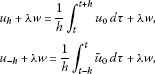where $w\in {X}_{2}$, λ is a real, ${\stackrel{˜}{u}}_{0}={u}_{0}$ if $t\ge 0$, and ${\stackrel{˜}{u}}_{0}=0$ if $t<0$. Thus the equality (3.16) is read as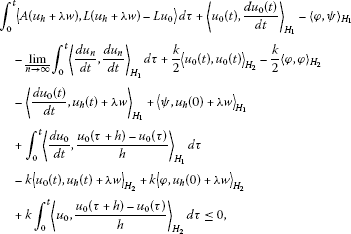(3.17)

and,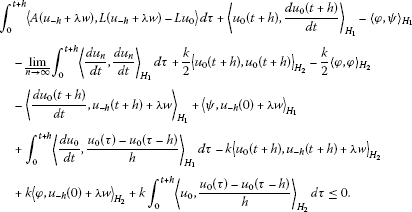(3.18)

In view of (3.17) and (3.18), we have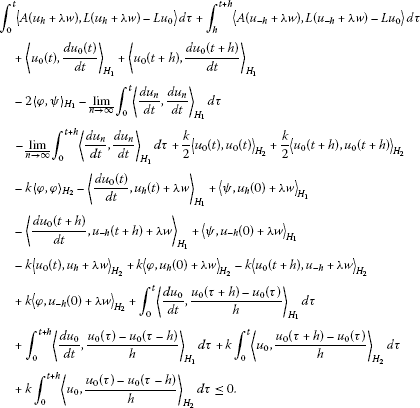(3.19)

We know that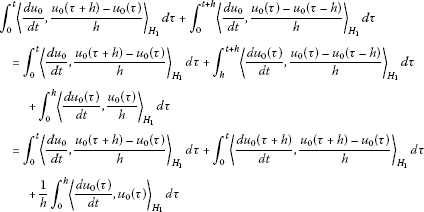and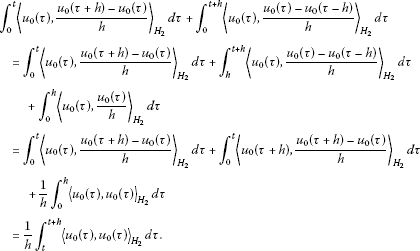Let $h\to {0}^{+}$. (3.19) can be read as

${\int }_{0}^{t}〈A\left({u}_{0}+\lambda w\right),\lambda Lw〉\phantom{\rule{0.2em}{0ex}}d\tau -{〈\frac{d{u}_{0}}{dt},\lambda w〉}_{{H}_{1}}-k{〈{u}_{0},\lambda w〉}_{{H}_{2}}+{〈\psi ,\lambda w〉}_{{H}_{1}}+k{〈\phi ,\lambda w〉}_{{H}_{2}}\le 0.$

According to (2.2) and (2.5), we obtain that

${\int }_{0}^{t}〈A\left({u}_{0}+\lambda w\right),Lw〉\phantom{\rule{0.2em}{0ex}}d\tau -{〈\frac{d{u}_{0}}{dt},Lw〉}_{H}-k{〈\mathcal{L}{u}_{0},Lw〉}_{H}+{〈\psi ,Lw〉}_{{H}_{1}}+k{〈\mathcal{L}\phi ,Lw〉}_{H}\le 0.$

Let $\lambda \to {0}^{+}$. It follows that

${\int }_{0}^{t}〈A\left({u}_{0}\right),Lw〉\phantom{\rule{0.2em}{0ex}}d\tau -{〈\frac{d{u}_{0}}{dt},Lw〉}_{H}-k{〈\mathcal{L}{u}_{0},Lw〉}_{H}+{〈\psi ,Lw〉}_{{H}_{1}}+k{〈\mathcal{L}\phi ,Lw〉}_{H}\le 0.$

Since $L:{X}_{2}\to {X}_{1}$ is dense, the above inequality can be rewritten as

${〈\frac{d{u}_{0}}{dt},v〉}_{H}+k{〈\mathcal{L}{u}_{0},v〉}_{H}={\int }_{0}^{t}〈A\left({u}_{0}\right),v〉\phantom{\rule{0.2em}{0ex}}d\tau +{〈\psi ,v〉}_{H}+k{〈\mathcal{L}\phi ,v〉}_{H},$

which implies that ${u}_{0}\in {W}^{1,\mathrm{\infty }}\left(\left(0,\mathrm{\infty }\right),{H}_{1}\right)\cap {W}^{1,2}\left(\left(0,\mathrm{\infty }\right),{H}_{2}\right)\cap {L}^{\mathrm{\infty }}\left(\left(0,\mathrm{\infty }\right),{X}_{2}\right)$ is a global weak solution of Eq. (2.4).

Secondly, we consider $G=A+B$. Let $v=\frac{d}{dt}L{u}_{n}$ in (3.12). In view of (2.2) and (3.1), it follows that

$\frac{1}{2}{\parallel \frac{d{u}_{n}}{dt}\parallel }_{{H}_{1}}^{2}+k{\int }_{0}^{t}{\parallel \frac{d{u}_{n}}{dt}\parallel }_{{H}_{2}}^{2}\phantom{\rule{0.2em}{0ex}}dt+F\left({u}_{n}\right)={\int }_{0}^{t}〈B\left({u}_{n}\right),\frac{d}{dt}L{u}_{n}〉\phantom{\rule{0.2em}{0ex}}dt+F\left({\phi }_{n}\right)+\frac{1}{2}{\parallel {\psi }_{n}\parallel }_{{H}_{1}}^{2}.$

From (3.3), we have

$\frac{1}{2}{\parallel \frac{d{u}_{n}}{dt}\parallel }_{{H}_{1}}^{2}+F\left({u}_{n}\right)+k{\int }_{0}^{t}{\parallel \frac{d{u}_{n}}{dt}\parallel }_{{H}_{2}}^{2}\phantom{\rule{0.2em}{0ex}}dt\le C{\int }_{0}^{t}\left[F\left({u}_{n}\right)+\frac{1}{2}{\parallel \frac{d{u}_{n}}{dt}\parallel }_{{H}_{1}}^{2}\right]\phantom{\rule{0.2em}{0ex}}dt+f\left(t\right),$
(3.20)

where $f\left(t\right)={\int }_{0}^{t}g\left(\tau \right)\phantom{\rule{0.2em}{0ex}}d\tau +\frac{1}{2}{\parallel \psi \parallel }_{{H}_{1}}^{2}+{sup}_{n}F\left({\phi }_{n}\right)$.

By using the Gronwall inequality, it follows that

$\frac{1}{2}{\parallel \frac{d{u}_{n}}{dt}\parallel }_{{H}_{1}}^{2}+F\left({u}_{n}\right)\le f\left(0\right){e}^{Ct}+{\int }_{0}^{t}f\left(\tau \right){e}^{C\left(t-\tau \right)}\phantom{\rule{0.2em}{0ex}}d\tau ,$
(3.21)

which implies that for all $0,

From (3.20) and (3.21), it follows that

Let

(3.22)

which implies that ${u}_{n}\to {u}_{0}$ in ${W}^{1,2}\left(\left(0,T\right),H\right)$ is uniformly weakly convergent from that ${H}_{2}\subset H$ is a compact imbedding.

The remaining part of the proof is same as assertion (1).

Lastly, assume (3.9) holds. Let $v=\frac{{d}^{2}{u}_{n}}{d{t}^{2}}$ in (3.12). It follows that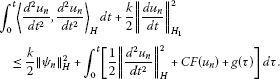From (3.21), the above inequality implies

(3.23)

We see that for all $0, $\left\{{u}_{n}\right\}\subset {W}^{2,2}\left(\left(0,T\right),H\right)$ is bounded. Thus $u\in {W}^{2,2}\left(\left(0,T\right),H\right)$. □

## 4 Main result

Now, we consider the nonlinear strongly damped plate or beam equation (1.1). Set

$F\left(x,y\right)={\int }_{0}^{y}f\left(x,z\right)\phantom{\rule{0.2em}{0ex}}dz.$
(4.1)

We assume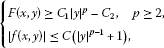(4.2)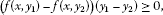(4.3)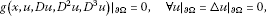(4.4)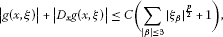(4.5)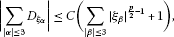(4.6)

where $\xi =\left\{{\xi }_{\alpha }||\alpha |\le 3\right\}$, ${\xi }_{\alpha }$ corresponds to ${D}^{\alpha }u$.

Theorem 4.1 Under the assumptions (4.1)-(4.6), if φ satisfies the bounded condition of Eq. (1.1), for$\left(\phi ,\psi \right)\in {W}^{4,p}\left(\mathrm{\Omega }\right)\cap {H}^{2}×{H}_{0}^{1}\left(\mathrm{\Omega }\right)$, then there exists a global strong solution for Eq. (1.1)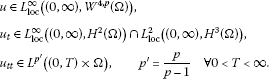Proof We introduce spatial sequences

$\begin{array}{c}X=\left\{u\in {C}^{\mathrm{\infty }}\left(\mathrm{\Omega }\right){|{\mathrm{△}}^{k}u|}_{\partial \mathrm{\Omega }}=0,k=0,1,2,\dots \right\},\hfill \\ {X}_{1}={L}^{p}\left(\mathrm{\Omega }\right),\phantom{\rule{2em}{0ex}}{X}_{2}=\left\{{W}^{4,p}\left(\mathrm{\Omega }\right){|u|}_{\partial \mathrm{\Omega }}=\mathrm{△}u{|}_{\partial \mathrm{\Omega }}=0\right\},\hfill \\ H={L}^{2}\left(\mathrm{\Omega }\right),\phantom{\rule{2em}{0ex}}{H}_{1}={H}^{2}\left(\mathrm{\Omega }\right)\cap {H}_{0}^{1}\left(\mathrm{\Omega }\right),\hfill \\ {H}_{2}=\left\{{H}^{3}\left(\mathrm{\Omega }\right){|u|}_{\partial \mathrm{\Omega }}=\mathrm{△}u{|}_{\partial \mathrm{\Omega }}=0\right\},\hfill \\ {H}_{3}=\left\{u\in {H}^{4m}\left(\mathrm{\Omega }\right){|u|}_{\partial \mathrm{\Omega }}=\cdots ={\mathrm{△}}^{2m-1}u{|}_{\partial \mathrm{\Omega }}\right\},\hfill \end{array}$

where the inner products of ${H}_{1}$, ${H}_{2}$ and ${H}_{3}$ are defined by

$\begin{array}{c}{〈u,v〉}_{{H}_{1}}={\int }_{\mathrm{\Omega }}\mathrm{△}u\mathrm{△}v\phantom{\rule{0.2em}{0ex}}dx,\phantom{\rule{2em}{0ex}}{〈u,v〉}_{{H}_{2}}={\int }_{\mathrm{\Omega }}▽\mathrm{△}u▽\mathrm{△}v\phantom{\rule{0.2em}{0ex}}dx,\hfill \\ {〈u,v〉}_{{H}_{3}}={\int }_{\mathrm{\Omega }}{\mathrm{△}}^{2m}u{\mathrm{△}}^{2m}v\phantom{\rule{0.2em}{0ex}}dx,\hfill \end{array}$

where $m\ge 1$ such that ${H}_{3}\subset {X}_{2}$ is an embedding.

Linear operator $\mathcal{L}:{X}_{2}\to {X}_{1}$ and $L:{X}_{2}\to {X}_{1}$ is defined by

$\mathcal{L}u=-\mathrm{△}u,\phantom{\rule{2em}{0ex}}Lu={\mathrm{△}}^{2}u.$

It is known that $\mathcal{L}$ and L satisfy (2.2), (2.3) and (2.5). Define $G=A+B:{X}_{2}\to {X}_{1}^{\ast }$ by

Let ${F}_{1}\left(u\right)={\int }_{\mathrm{\Omega }}F\left(x,\mathrm{△}u\right)\phantom{\rule{0.2em}{0ex}}dx$, where F is the same as in (4.2). We get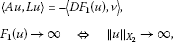which implies conditions (A1), (A2) of Theorem 3.1.

From (4.3), we have

$〈D{F}_{1}\left({u}_{1}\right)-D{F}_{1}\left({u}_{2}\right),{u}_{1}-{u}_{2}〉\ge 0.$

From (4.5) and (4.6), we obtain that $B:{X}_{2}\to {X}_{1}^{\ast }$ is a compact operator. Then, B satisfies (3.6) and (3.7).

We will show (3.3) as follows. From (4.4) and (4.5), for $\mathrm{\forall }u,v\in X$, it follows that

$\begin{array}{rcl}|〈Bu,Lv〉|& =& {\int }_{\mathrm{\Omega }}|g\left(x,u,Du,{D}^{2}u,{D}^{3}u\right){\mathrm{△}}^{2}v|\phantom{\rule{0.2em}{0ex}}dx\\ =& {\int }_{\mathrm{\Omega }}|\mathrm{\nabla }g\left(x,u,Du,{D}^{2}u,{D}^{3}u\right)\cdot ▽\mathrm{△}v|\phantom{\rule{0.2em}{0ex}}dx\\ \le & \frac{k}{2}{\int }_{\mathrm{\Omega }}{|▽\mathrm{△}v|}^{2}\phantom{\rule{0.2em}{0ex}}dx+\frac{2}{k}{\int }_{\mathrm{\Omega }}{|▽g\left(x,u,Du,{D}^{2}u,{D}^{3}u\right)|}^{2}\phantom{\rule{0.2em}{0ex}}dx\\ \le & \frac{k}{2}{\parallel v\parallel }_{{H}_{2}}^{2}+C{\int }_{\mathrm{\Omega }}\left[{|{D}_{x}g|}^{2}+\sum _{i=1}^{n}\sum _{|\alpha |\le 3}{|{D}_{{\zeta }_{\alpha }}g|}^{2}{|{D}_{i}{D}^{\alpha }u|}^{2}\right]\phantom{\rule{0.2em}{0ex}}dx\\ \le & \frac{k}{2}{\parallel v\parallel }_{{H}_{2}}^{2}+C{\int }_{\mathrm{\Omega }}\left[\sum _{|\alpha |\le 4}{|{D}^{\alpha }u|}^{p}+1\right]\phantom{\rule{0.2em}{0ex}}dx,\end{array}$

which implies condition (A3) of Theorem 3.1. From Theorem 3.1, Eq. (1.1) has a solution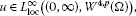(4.7)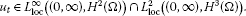(4.8)

Lastly, we show that ${u}^{″}\in {L}^{{p}^{\prime }}\left(\mathrm{\Omega }×\left(0,T\right)\right)$. By Definition 2.1, u satisfies

$\begin{array}{rcl}{\int }_{\mathrm{\Omega }}{u}_{t}\left(x,t\right)v\phantom{\rule{0.2em}{0ex}}dx+{\int }_{\mathrm{\Omega }}u\left(x,t\right)v\phantom{\rule{0.2em}{0ex}}dx& =& {\int }_{0}^{t}{\int }_{\mathrm{\Omega }}\left[f\left(x,\mathrm{△}u\right)+g\left(x,u,Du,{D}^{2}u,{D}^{3}u\right)v\right]\phantom{\rule{0.2em}{0ex}}dx\phantom{\rule{0.2em}{0ex}}dt\\ +{\int }_{\mathrm{\Omega }}\psi v\phantom{\rule{0.2em}{0ex}}dx+{\int }_{\mathrm{\Omega }}\phi v\phantom{\rule{0.2em}{0ex}}dx,\phantom{\rule{1em}{0ex}}\mathrm{\forall }v\in {L}^{p}\left(\mathrm{\Omega }\right).\end{array}$

Then, for any $h>0$, it follows that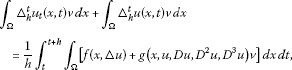(4.9)

where ${\mathrm{△}}_{h}^{t}u=\frac{1}{h}\left(u\left(t+h\right)-u\left(t\right)\right)$. Let $v={|{\mathrm{△}}_{h}^{t}{u}_{t}|}^{{p}^{\prime }-2}{\mathrm{△}}_{h}^{t}{u}_{t}$. From (4.9), we have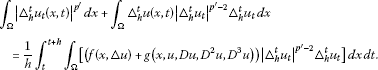Then, it follows that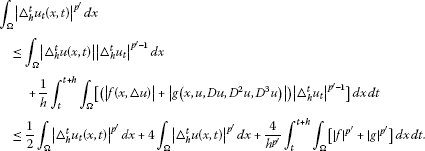From (4.2) and (4.5), we have

$\begin{array}{c}{\int }_{0}^{T}{\int }_{\mathrm{\Omega }}{|\frac{{u}_{t}\left(x,t+h\right)-{u}_{t}\left(x,t\right)}{h}|}^{{p}^{\prime }}\phantom{\rule{0.2em}{0ex}}dx\phantom{\rule{0.2em}{0ex}}dt\hfill \\ \phantom{\rule{1em}{0ex}}\le C{\int }_{0}^{T}{\int }_{\mathrm{\Omega }}{|{\mathrm{△}}_{h}^{t}u\left(x,t\right)|}^{{p}^{\prime }}\phantom{\rule{0.2em}{0ex}}dx\phantom{\rule{0.2em}{0ex}}dt\hfill \\ \phantom{\rule{2em}{0ex}}+C{\int }_{0}^{T}{\int }_{t}^{t+h}{\int }_{\mathrm{\Omega }}\left[{|f|}^{{p}^{\prime }}+{|g|}^{{p}^{\prime }}\right]\phantom{\rule{0.2em}{0ex}}dx\phantom{\rule{0.2em}{0ex}}d\tau \phantom{\rule{0.2em}{0ex}}dt\hfill \\ \phantom{\rule{1em}{0ex}}\le C{\int }_{0}^{T}{\int }_{\mathrm{\Omega }}{|{u}_{t}\left(x,t\right)|}^{\frac{p}{p-1}}\phantom{\rule{0.2em}{0ex}}dx\phantom{\rule{0.2em}{0ex}}dt\hfill \\ \phantom{\rule{2em}{0ex}}+C{\int }_{0}^{T}{\int }_{\mathrm{\Omega }}\left[{\left({|\mathrm{△}u|}^{p-1}+1\right)}^{{p}^{\prime }}+{\left(\sum _{|\alpha |\le 3}{|{D}^{\alpha }u|}^{\frac{p}{2}}+1\right)}^{{p}^{\prime }}\right]\phantom{\rule{0.2em}{0ex}}dx\phantom{\rule{0.2em}{0ex}}dt\hfill \\ \phantom{\rule{1em}{0ex}}\le C{\int }_{0}^{T}{\int }_{\mathrm{\Omega }}{|{u}_{t}\left(x,t\right)|}^{\frac{p}{p-1}}\phantom{\rule{0.2em}{0ex}}dx\phantom{\rule{0.2em}{0ex}}dt+C{\int }_{0}^{T}{\int }_{\mathrm{\Omega }}\left[{|\mathrm{△}u|}^{p}+\sum _{|\alpha |\le 3}{|{D}^{\alpha }u|}^{\frac{{p}^{2}}{2\left(p-1\right)}}+1\right]\phantom{\rule{0.2em}{0ex}}dx\phantom{\rule{0.2em}{0ex}}dt.\hfill \end{array}$

By using the Sobolev embedding theorem, it follows that from (4.7) and (4.8) the right of the above inequality is bounded. Then, ${u}_{tt}$ exists almost everywhere in $\mathrm{\Omega }×\left(0,T\right)$, and ${u}_{tt}\in {L}^{{p}^{\prime }}\left(\mathrm{\Omega }×\left(0,T\right)\right)$, $\mathrm{\forall }0. □

## References

1. Lazer AC, McKenna PJ: Large-amplitude periodic oscillations in suspension bridges: some new connections with nonlinear analysis. SIAM Rev. 1990, 32: 537-578. 10.1137/1032120

2. Lazer AC, McKenna PJ: Large scale oscillatory behavior in loaded asymmetric systems. Ann. Inst. Henri Poincaré, Anal. Non Linéaire 1987, 4: 243-274.

3. McKenna PJ, Walter W: Nonlinear oscillations in a suspension bridges. Arch. Ration. Mech. Anal. 1987, 98: 167-177.

4. McKenna PJ, Walter W: Traveling waves in a suspension bridge. SIAM J. Appl. Math. 1990, 50: 702-715.

5. Ahmed NU, Biswas SK: Mathematical modeling and control of large space structures with multiple appendages. Math. Comput. Model. 1988, 10: 891-900. 10.1016/0895-7177(88)90181-1

6. Ahmed NU, Harbi H: Mathematical analysis of dynamical models of suspension bridges. SIAM J. Appl. Math. 1998, 58: 853-874. 10.1137/S0036139996308698

7. Krol MS: On a Galerkin-averaging method for weakly nonlinear wave equations. Math. Methods Appl. Sci. 1989, 11: 649-664. 10.1002/mma.1670110506

8. van Horssen WT: An asymptotic theory for a class of initial-boundary value problems for weakly nonlinear wave equations with an application to a model of the galloping oscillations of overhead transmission lines. SIAM J. Appl. Math. 1988, 48: 1227-1243. 10.1137/0148075

9. Medeiros LA: On a new class of nonlinear wave equation. J. Math. Anal. Appl. 1979, 69: 252-262. 10.1016/0022-247X(79)90192-6

10. Nakao M: Decay of solutions of some nonlinear evolution equations. J. Math. Anal. Appl. 1977, 60: 542-549. 10.1016/0022-247X(77)90040-3

11. Nishihara K: Exponentially decay of solutions of some quasilinear hyperbolic equations with linear damping. Nonlinear Anal. 1984, 8: 623-636. 10.1016/0362-546X(84)90007-5

12. Patcheu SK: On a global solution and asymptotic behaviour for the generalized damped extensible beam equation. J. Differ. Equ. 1997, 135: 299-314. 10.1006/jdeq.1996.3231

13. Pereira DC: Existence uniqueness and asymptotic behaviour for solutions of the nonlinear beam equation. Nonlinear Anal. 1990, 14: 613-623. 10.1016/0362-546X(90)90041-E

14. Kim JA, Lee K: Energy decay for the strongly damped nonlinear beam equation and its applications in moving boundary. Acta Appl. Math. 2010, 109: 507-525. 10.1007/s10440-008-9330-3

15. Ma T, Wang SH World Sci. Ser. Nonlinear Sci. Ser. A Monogr. Treatises 53. In Bifurcation Theory and Applications. World Scientific, Singapore; 2005.

16. Ma T, Wang SH: Stability and Bifurcation of Nonlinear Evolution Equations. Science Press, China; 2007. in Chinese

17. Ma T, Wang SH: Phase Transition Dynamics in Nonlinear Sciences. New York, Springer; 2012.

18. Ma T: Theories and Methods for Partial Differential Equations. Science Press, China; 2011. in Chinese

## Acknowledgement

The authors are very grateful to the anonymous referees whose careful reading of the manuscript and valuable comments enhanced the presentation of the manuscript. Supported by the National Natural Science Foundation of China (NO. 11071177), the NSF of Sichuan Science and Technology Department of China (NO. 2010JY0057) and the NSF of Sichuan Education Department of China (NO. 11ZA102).

## Author information

Authors

### Corresponding author

Correspondence to Hong Luo.

### Competing interests

The authors declare that they have no competing interests.

### Authors’ contributions

All authors typed, read and approved the final manuscript.

## Rights and permissions

Reprints and Permissions

Luo, H., Li, Lm. & Ma, T. Existence of solutions to strongly damped plate or beam equations. Bound Value Probl 2012, 76 (2012). https://doi.org/10.1186/1687-2770-2012-76

• Accepted:

• Published:

• DOI: https://doi.org/10.1186/1687-2770-2012-76

### Keywords

• existence
• solution
• plate
• beam
• strongly damped